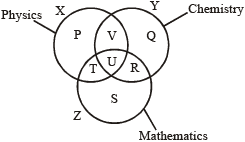### Venn Diagram Test

1. Directions (Q. 1-5) : In the following diagram, the circle represents college Professors, the triangle stands for surgical Specialists, and medical Specialists are represented by the rectangle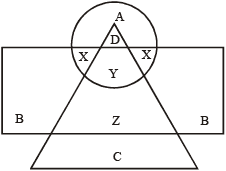College professors who are also surgical Specialists are represented by:

2. Directions (Q. 1-5) : In the following diagram, the circle represents college Professors, the triangle stands for surgical Specialists, and medical Specialists are represented by the rectangleSurgical Specialists who are also Medical Specialists but not Professors are represented by:

3. Directions (Q. 1-5) : In the following diagram, the circle represents college Professors, the triangle stands for surgical Specialists, and medical Specialists are represented by the rectangleC represents:

4. Directions (Q. 1-5) : In the following diagram, the circle represents college Professors, the triangle stands for surgical Specialists, and medical Specialists are represented by the rectangleB represents :

5. Directions (Q. 1-5) : In the following diagram, the circle represents college Professors, the triangle stands for surgical Specialists, and medical Specialists are represented by the rectangleCollege Professors who are also Medical Specialists are represented by:

6. Directions (Q. 6-10) : Answer these questions based on the diagram given below.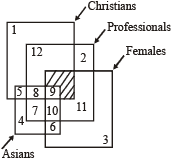Asian Non-Christian females who are Professionals are represented by:

7. Directions (Q. 6-10) : Answer these questions based on the diagram given below.Asian females who are neither professional nor Christians are denoted by:

8. Directions (Q. 6-10) : Answer these questions based on the diagram given below.Non-Asian professional Christian male are represented by:

9. Directions (Q. 6-10) : Answer these questions based on the diagram given below.Christian females who are non-professional and Asian are represented by

10. Directions (Q. 6-10) : Answer these questions based on the diagram given below.11. Directions (Q. 11-14) : In the following figure, the smaller triangle represents teachers; the big triangle represents politicians; circle represents graduates and rectangle represents members of Parliament. Different regions are being represented by letters of English alphabet.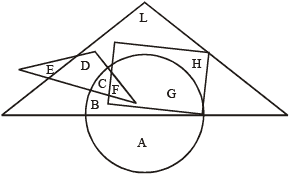Which among the following regions represent the graduates or teachers but not politicians?

12. Directions (Q. 11-14) : In the following figure, the smaller triangle represents teachers; the big triangle represents politicians; circle represents graduates and rectangle represents members of Parliament. Different regions are being represented by letters of English alphabet.Which among the following regions represent the graduate politicians but not the members of Parliament?

13. Directions (Q. 11-14) : In the following figure, the smaller triangle represents teachers; the big triangle represents politicians; circle represents graduates and rectangle represents members of Parliament. Different regions are being represented by letters of English alphabet.Which among the following regions represent the politicians, who are neither teachers nor graduates?

14. Directions (Q. 11-14) : In the following figure, the smaller triangle represents teachers; the big triangle represents politicians; circle represents graduates and rectangle represents members of Parliament. Different regions are being represented by letters of English alphabet.Which among the following regions represent the members of parliament, who are graduate as well as a teacher?

15. Directions (Qs 15- 19) : In the following figure, rectangle, square, circle and triangle represent the regions of wheat, gram, maize and rice cultivation, respectively. On the basis of the figure, answer the following questions :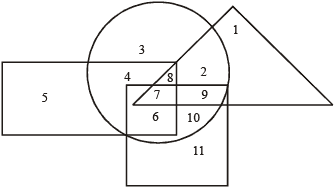Which area is cultivated by all the four commodities?

16. Directions (Qs 15- 19) : In the following figure, rectangle, square, circle and triangle represent the regions of wheat, gram, maize and rice cultivation, respectively. On the basis of the figure, answer the following questions :Which area is cultivated by wheat and maize only?

17. Directions (Qs 15- 19) : In the following figure, rectangle, square, circle and triangle represent the regions of wheat, gram, maize and rice cultivation, respectively. On the basis of the figure, answer the following questions :Which area is cultivated by rice only?

18. Directions (Qs 15- 19) : In the following figure, rectangle, square, circle and triangle represent the regions of wheat, gram, maize and rice cultivation, respectively. On the basis of the figure, answer the following questions :Which area is cultivated by maize only?

19. Directions (Qs 15- 19) : In the following figure, rectangle, square, circle and triangle represent the regions of wheat, gram, maize and rice cultivation, respectively. On the basis of the figure, answer the following questions :Which area is cultivated by rice and maize and nothing else?

20. Directions : (Qs.20-24) : In the following diagram, three classes of population are represented by three figures. The triangle represents the school teachers, the square represents the married persons and the circle represents the persons living in joint families.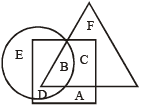Married persons living in joint families but not working as teachers are represented by:

21. Directions : (Qs.20-24) : In the following diagram, three classes of population are represented by three figures. The triangle represents the school teachers, the square represents the married persons and the circle represents the persons living in joint families.Persons who live in joint families, are unmarried and who do not work as teachers are represented by:

22. Directions : (Qs.20-24) : In the following diagram, three classes of population are represented by three figures. The triangle represents the school teachers, the square represents the married persons and the circle represents the persons living in joint families.Married teachers living in joint families are represented by:

23. Directions : (Qs.20-24) : In the following diagram, three classes of population are represented by three figures. The triangle represents the school teachers, the square represents the married persons and the circle represents the persons living in joint families.School teachers who are married but do not live in joint families are represented by

24. Directions : (Qs.20-24) : In the following diagram, three classes of population are represented by three figures. The triangle represents the school teachers, the square represents the married persons and the circle represents the persons living in joint families.School teachers who are neither married nor do live in joint families are represented by:

25. Directions (Q.25-28) : Refer to the following Venn diagram:The number of students who took any three of the above subjects was:

26. Directions (Q.25-28) : Refer to the following Venn diagram:The number of students in total, who took History or Mathematics or Science, was:

27. Directions (Q.25-28) : Refer to the following Venn diagram:The number of students who took both History and Geography among other subjects was:

28. Directions (Q.25-28) : Refer to the following Venn diagram:Which subject was taken by the largest number of students?

29. In the given figure, the triangle represents girls, the square represents sports persons and the circle represents coaches. The portion in the figure which represents girls, who are sports persons but not coaches is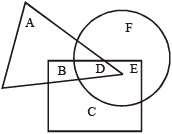30. If Tall men is equivalent to circle, Army men to triangle and Strong men to square. In figure, different regions are represented by different numbers from 1 to 7. Indicate which number will represent strong army men?31. The diagram below represents the students who study Physics, Chemistry and Mathematics. Study the diagram and identify the region which represents the students who study Physics and Mathematics but not Chemistry.